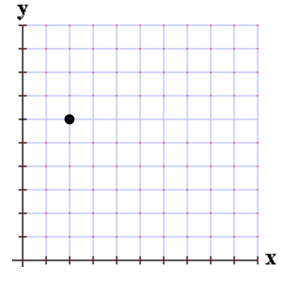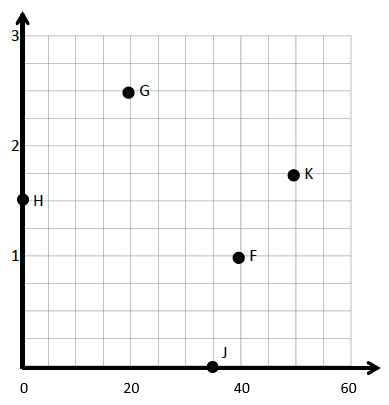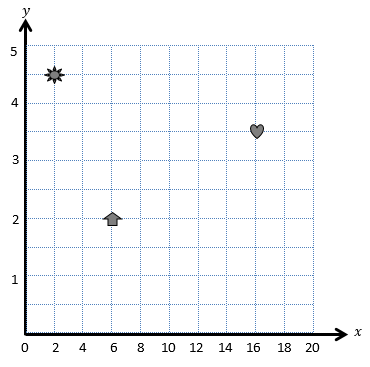# Patterns and the Coordinate Plane

## Objective

Construct a coordinate plane with non-unit intervals and use it to plot and identify points.

## Common Core Standards

### Core Standards

?

• 5.G.A.1 — Use a pair of perpendicular number lines, called axes, to define a coordinate system, with the intersection of the lines (the origin) arranged to coincide with the 0 on each line and a given point in the plane located by using an ordered pair of numbers, called its coordinates. Understand that the first number indicates how far to travel from the origin in the direction of one axis, and the second number indicates how far to travel in the direction of the second axis, with the convention that the names of the two axes and the coordinates correspond (e.g., x-axis and x-coordinate, y-axis and y-coordinate).

?

• 2.MD.B.6

• 3.NF.A.2

## Criteria for Success

?

1. Understand that a coordinate grid can have intervals that are not equal to one and that the length of each interval is called the scale of a graph.
2. Given a point in a coordinate plane with non-unit intervals, determine its location and write it as an ordered pair.
3. Given the coordinates of a point, plot it on a coordinate plane with non-unit intervals.

## Tips for Teachers

?

• You may decide to adapt this lesson and the next one, depending on pacing as well as students’ readiness for these lessons. These lessons help to prepare students for Question 5 on the Unit Assessment, but because students can construct a coordinate graph with a scale of 1 on both axes on the provided grid, they aren’t entirely necessary. Of the released items from the main standardized tests (PARCC, SBAC, NY Regents, MCAS), there is only one item that relies on these skills. Thus, it’s safe to assume that it’s not an expected skill at this grade level. If you do decide that students don’t need these skills, it will probably be a good idea to still keep time in the unit to ensure students have a deep understanding of the coordinate plane. Thus, you could adapt them as follows:
• Lesson 3: Cut the Anchor Tasks and have the bulk of the lesson be spent playing Battleship strictly on a coordinate plane where both axes have a scale of 1.
• Lesson 4: Modify the Anchor Tasks and Problem Set/Homework so that students are still expected to construct a coordinate plane from scratch and plot various points, but limit the coordinates to within a reasonable number, e.g., 20. You could focus on the axes not needing to exceed the largest x- and y-coordinate (e.g., in one task the axes may only need to go to 5 but in another need to go to 20).
• While the work of Lessons 1 and 2 required students to attend to precision to plot points and identify the location of points in a coordinate grid, that practice is called upon even more so as students deal with non-interval units in Lesson 3 (MP.6).
• For the problem set, make sure students understand the rules to Battleship. You might decide to model a round of play just so students get the hang of it. You also might decide to give students some constraints on the axes they choose, just to ensure they’re practicing today’s newly acquired skill of plotting and naming points on a coordinate grid with non-unit intervals. Have students play multiple rounds, changing the scales on one or both axes with each new round.
• For the homework, students will play Battleship with a family member. Send students home with an extra copy of the Problem Set or with grid paper.

### Lesson Materials

• Markers or crayons (2 per student) — Need red and black

#### Fishtank Plus

• Problem Set
• Student Handout Editor
• Vocabulary Package

?

### Problem 1

Heidi and Beatriz are both trying to determine the location of the following point.Heidi says that the point is located at (2, 18). Beatriz says that the point is located at (1, 6). How could both Heidi and Beatriz be correct? Explain.

### Problem 2

1. Determine the coordinates of all the points on the following coordinate plane.1. Plot the following points on the coordinate plane above:

Point L: (40, 3)                    Point M${(10, {1\over2})}$                    Point N${(55, 2{3\over12})}$

#### References

EngageNY Mathematics Grade 5 Mathematics > Module 6 > Topic A > Lesson 3Fluency Practice

Grade 5 Mathematics > Module 6 > Topic A > Lesson 3 of the New York State Common Core Mathematics Curriculum from EngageNY and Great Minds. © 2015 Great Minds. Licensed by EngageNY of the New York State Education Department under the CC BY-NC-SA 3.0 US license. Accessed Dec. 2, 2016, 5:15 p.m..

Modified by Fishtank Learning, Inc.

## Problem Set & Homework

### Discussion of Problem Set

• What was your strategy in choosing where to set up your fleet? Did it work? What would you do differently next time?
• How did you decide where to make your attack shots?
• When you hit an opponent’s ship, how did you plan your next shot?
• What did your opponent do that seemed to work well for him?
• What could be done to the coordinate plane to make the game easier or more challenging?
• How did today’s game strengthen your understanding of the coordinate plane?
• Do you think coordinate pairs are actually used in battle? Why or why not?

?

Use the diagram below for each of the following:a.   Name the coordinates of each shape.

Sun: _______________

Arrow: ______________

Heart: ______________

b.   Plot a square at ${(3,2)}$ and a triangle at ${\left(4, 1{1\over2}\right)}$.

#### References

EngageNY Mathematics Grade 5 Mathematics > Module 6 > Topic A > Lesson 2Exit Ticket

Grade 5 Mathematics > Module 6 > Topic A > Lesson 2 of the New York State Common Core Mathematics Curriculum from EngageNY and Great Minds. © 2015 Great Minds. Licensed by EngageNY of the New York State Education Department under the CC BY-NC-SA 3.0 US license. Accessed Dec. 2, 2016, 5:15 p.m..

Modified by Fishtank Learning, Inc.